# For the reaction 2802(g) + O2(g) -2503(g) AH° = -197.8 kJ and AS° = -187.9 J/K...For the reaction 2802(g) + O2(g) -2503(g) AH° = -197.8 kJ and AS° = -187.9 J/K The equilibrium constant for this reaction at 277.0 K is Assume that AH° and ASº are independent of temperature.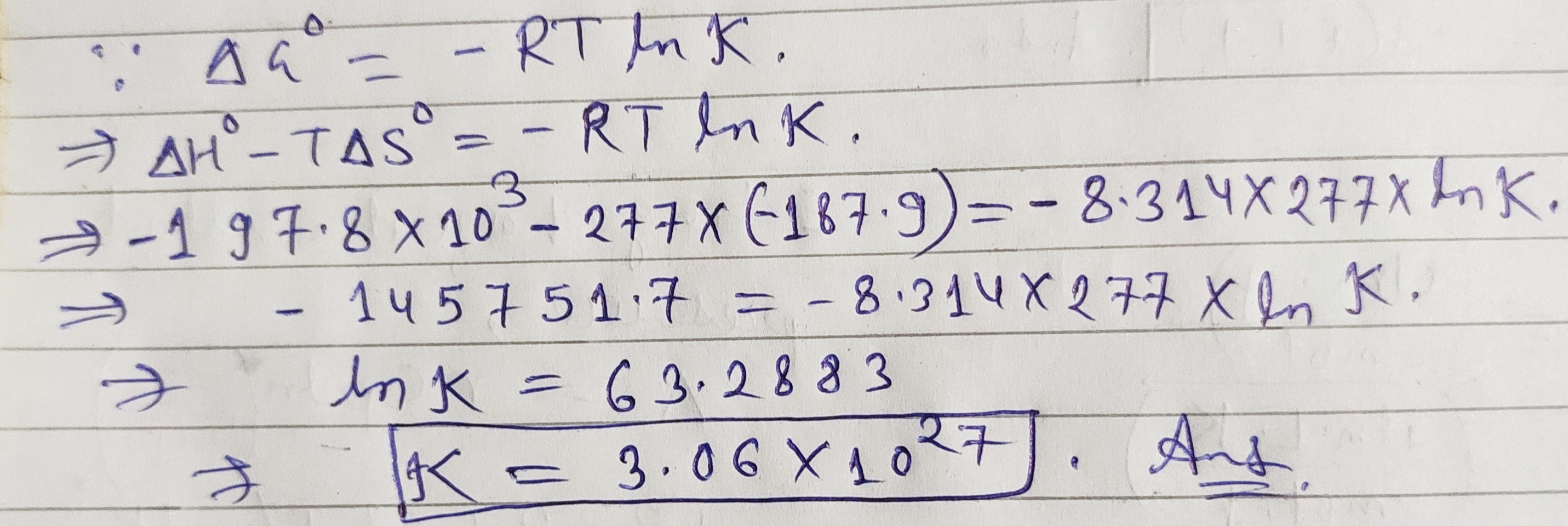#### Earn Coin

Coins can be redeemed for fabulous gifts.

Similar Homework Help Questions
• ### For the reaction 2802(g) + O2(g)—>2503(g) AH° = -197.8 kJ and AS° = -187.9 J/K The...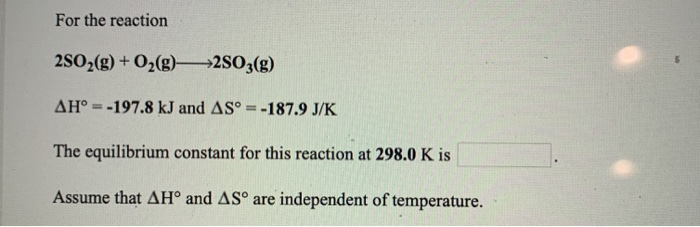For the reaction 2802(g) + O2(g)—>2503(g) AH° = -197.8 kJ and AS° = -187.9 J/K The equilibrium constant for this reaction at 298.0 K is Assume that AHⓇ and AS™ are independent of temperature.

• ### 11. For the reaction Fe2O3(s) + 3H2(g) +2Fe(s) + 3H2O(g) AH° = 98.8 kJ and AS°...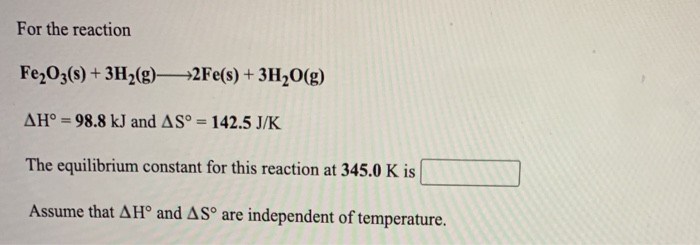11. For the reaction Fe2O3(s) + 3H2(g) +2Fe(s) + 3H2O(g) AH° = 98.8 kJ and AS° = 142.5 J/K The equilibrium constant for this reaction at 345.0 K is Assume that AHⓇ and AS are independent of temperature. For the reaction 2502(g) + O2(g) +2503(g) AH° = -197.8 kJ and AS° = -187.9 J/K The equilibrium constant for this reaction at 272.0 K is Assume that AH° and AS are independent of temperature.

• ### For the reaction N2(g) + O2(g)->2NO(g) AH° = 180.6 kJ and AS = 24.9 J/K The...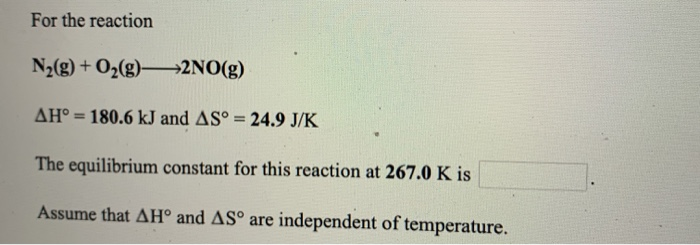For the reaction N2(g) + O2(g)->2NO(g) AH° = 180.6 kJ and AS = 24.9 J/K The equilibrium constant for this reaction at 267.0 K is Assume that AHⓇ and ASº are independent of temperature.

• ### For the reaction 4HCl(g) + O2(g)—>2H2O(g) + 2C12(g) AH = -114.4 kJ and AS°=-128.9 J/K The...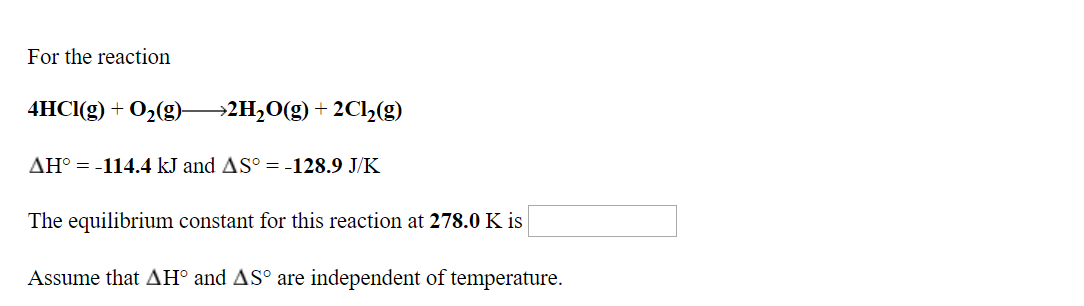For the reaction 4HCl(g) + O2(g)—>2H2O(g) + 2C12(g) AH = -114.4 kJ and AS°=-128.9 J/K The equilibrium constant for this reaction at 278.0 K is Assume that AH and ASº are independent of temperature.

• ### For the reaction N2(g) + O2(g)2NO(g) AH° - 180.6 kJ and AS - 24.9 J/K The...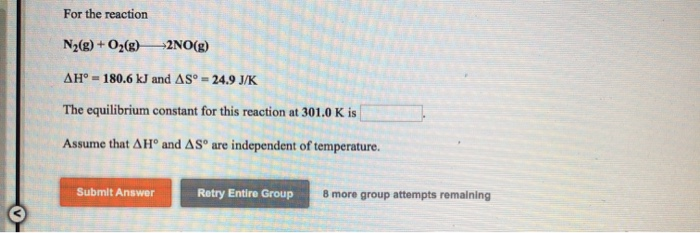For the reaction N2(g) + O2(g)2NO(g) AH° - 180.6 kJ and AS - 24.9 J/K The equilibrium constant for this reaction at 301.0K is Assume that A Hº and AS are independent of temperature. Submit Answer Retry Entire Group 8 more group attempts remaining

• ### For the reaction 2NO(g) + O2(g) —>2NO2(g) AH° = -114.2 kJ and AS° = -146.5 J/K...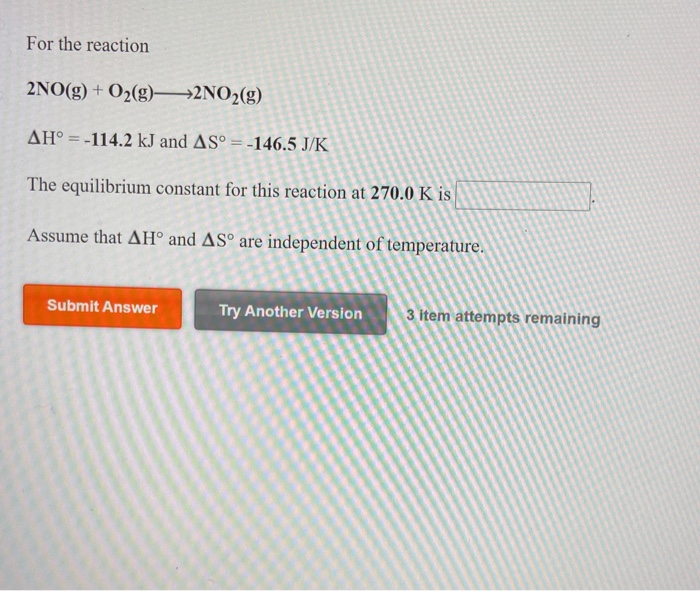For the reaction 2NO(g) + O2(g) —>2NO2(g) AH° = -114.2 kJ and AS° = -146.5 J/K The equilibrium constant for this reaction at 270.0 K is Assume that AH° and AS are independent of temperature. Submit Answer Try Another Version 3 item attempts remaining elefchces to access important values if needed for this question, Consider the reaction 2CO2(g) + 5H2(g)CH2(g) + 4H2O(g) Using the standard thermodynamic data in the tables linked above, calculate AG for this reaction at 298.15K if...

• ### For the reaction 4HCl(g) + O2(g)— *2H2O(g) + 2Cl2(9) AH' = -114.4 kJ and AS° =...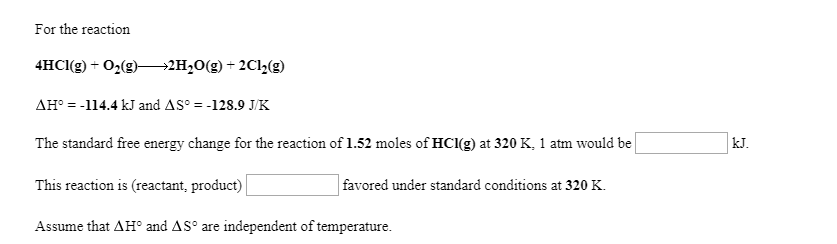For the reaction 4HCl(g) + O2(g)— *2H2O(g) + 2Cl2(9) AH' = -114.4 kJ and AS° = -128.9 J/K The standard free energy change for the reaction of 1.52 moles of HCI(g) at 320 K, 1 atm would be This reaction is (reactant, product) favored under standard conditions at 320 K. Assume that AH and AS are independent of temperature. For the reaction 2 SO2(g) +02(9) —2 SO3(9) AG° = -144.4 kJ and AS° = -187.9 J/K at 284 K and...

• ### For the reaction Fe(s) + 2HCl(aq) FeCl2(5) + H2(g) AH° = -7.4 kJ and AS° =...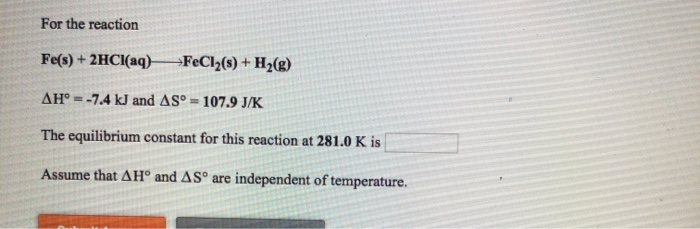For the reaction Fe(s) + 2HCl(aq) FeCl2(5) + H2(g) AH° = -7.4 kJ and AS° = 107.9 J/K The equilibrium constant for this reaction at 281.0 K is Assume that AHº and ASº are independent of temperature.

• ### 2NO(g)02(g)2NO2(g) AH° =-114.2 kJ and AS° =-146.5 J/K The equilibrium constant for this reaction at 321.0...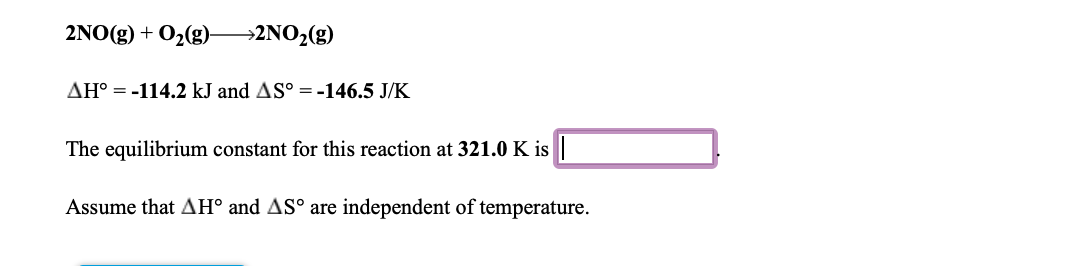2NO(g)02(g)2NO2(g) AH° =-114.2 kJ and AS° =-146.5 J/K The equilibrium constant for this reaction at 321.0 K is Assume that AH° and AS° are independent of temperature

• ### Given the following data: 3 s(s) + 2O2(9) S03(9) AH = -395.2 kJ 2802(g) + O2(g)...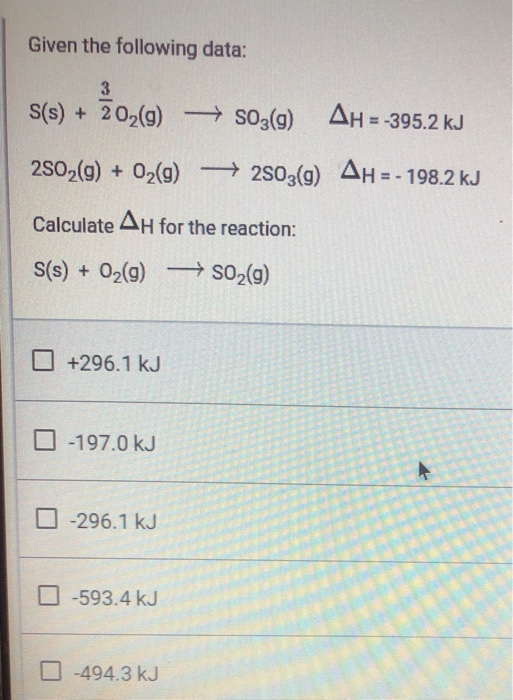Given the following data: 3 s(s) + 2O2(9) S03(9) AH = -395.2 kJ 2802(g) + O2(g) +2503(9) AH = - 198.2 kJ Calculate AH for the reaction: S(s) + O2(g) → SO2(g) +296.1 kJ -197.0 kJ -296.1 kJ -593.4 kJ -494.3 kJ